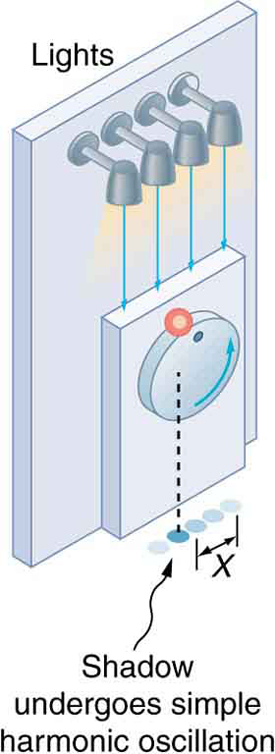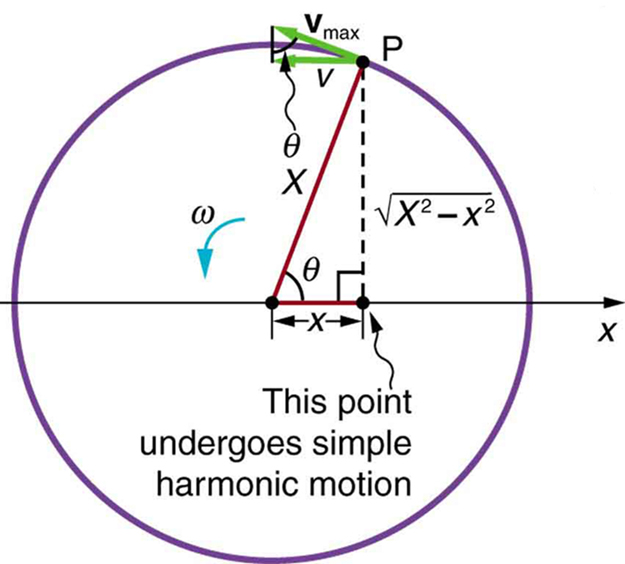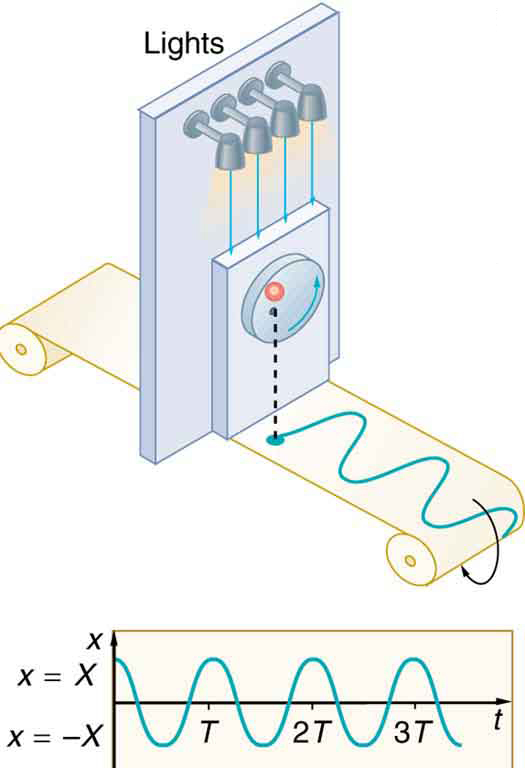# 16.6 Uniform circular motion and simple harmonic motion

 Page 1 / 1
• Compare simple harmonic motion with uniform circular motion.The horses on this merry-go-round exhibit uniform circular motion. (credit: Wonderlane, Flickr)

There is an easy way to produce simple harmonic motion by using uniform circular motion. [link] shows one way of using this method. A ball is attached to a uniformly rotating vertical turntable, and its shadow is projected on the floor as shown. The shadow undergoes simple harmonic motion. Hooke’s law usually describes uniform circular motions ( $\omega$ constant) rather than systems that have large visible displacements. So observing the projection of uniform circular motion, as in [link] , is often easier than observing a precise large-scale simple harmonic oscillator. If studied in sufficient depth, simple harmonic motion produced in this manner can give considerable insight into many aspects of oscillations and waves and is very useful mathematically. In our brief treatment, we shall indicate some of the major features of this relationship and how they might be useful.The shadow of a ball rotating at constant angular velocity ω size 12{ω} {} on a turntable goes back and forth in precise simple harmonic motion.

[link] shows the basic relationship between uniform circular motion and simple harmonic motion. The point P travels around the circle at constant angular velocity $\omega$ . The point P is analogous to an object on the merry-go-round. The projection of the position of P onto a fixed axis undergoes simple harmonic motion and is analogous to the shadow of the object. At the time shown in the figure, the projection has position $x$ and moves to the left with velocity $v$ . The velocity of the point P around the circle equals ${\overline{v}}_{\text{max}}$ .The projection of ${\overline{v}}_{\text{max}}$ on the $x$ -axis is the velocity $v$ of the simple harmonic motion along the $x$ -axis.A point P moving on a circular path with a constant angular velocity ω size 12{ω} {} is undergoing uniform circular motion. Its projection on the x-axis undergoes simple harmonic motion. Also shown is the velocity of this point around the circle, v ¯ max size 12{ {overline {v}} rSub { size 8{"max"} } } {} , and its projection, which is v size 12{v} {} . Note that these velocities form a similar triangle to the displacement triangle.

To see that the projection undergoes simple harmonic motion, note that its position $x$ is given by

$x=X\phantom{\rule{0.25em}{0ex}}\text{cos}\phantom{\rule{0.25em}{0ex}}\theta \text{,}$

where $\theta =\omega t$ , $\omega$ is the constant angular velocity, and $X$ is the radius of the circular path. Thus,

$x=X\phantom{\rule{0.25em}{0ex}}\text{cos}\phantom{\rule{0.25em}{0ex}}\omega t.$

The angular velocity $\omega$ is in radians per unit time; in this case $2\pi$ radians is the time for one revolution $T$ . That is, $\omega =2\pi /T$ . Substituting this expression for $\omega$ , we see that the position $x$ is given by:

$x\left(t\right)=\text{cos}\left(\frac{2\pi t}{T}\right).$

This expression is the same one we had for the position of a simple harmonic oscillator in Simple Harmonic Motion: A Special Periodic Motion . If we make a graph of position versus time as in [link] , we see again the wavelike character (typical of simple harmonic motion) of the projection of uniform circular motion onto the $x$ -axis.The position of the projection of uniform circular motion performs simple harmonic motion, as this wavelike graph of x size 12{x} {} versus t size 12{x} {} indicates.

Now let us use [link] to do some further analysis of uniform circular motion as it relates to simple harmonic motion. The triangle formed by the velocities in the figure and the triangle formed by the displacements ( $X,\phantom{\rule{0.25em}{0ex}}x,\phantom{\rule{0.25em}{0ex}}$ and $\sqrt{{X}^{2}-{x}^{2}}$ ) are similar right triangles. Taking ratios of similar sides, we see that

$\frac{v}{{v}_{\text{max}}}=\frac{\sqrt{{X}^{2}-{x}^{2}}}{X}=\sqrt{1-\frac{{x}^{2}}{{X}^{2}}}.$

We can solve this equation for the speed $v$ or

$v={v}_{\text{max}}\sqrt{1-\frac{{x}^{2}}{{X}^{2}}}.$

This expression for the speed of a simple harmonic oscillator is exactly the same as the equation obtained from conservation of energy considerations in Energy and the Simple Harmonic Oscillator .You can begin to see that it is possible to get all of the characteristics of simple harmonic motion from an analysis of the projection of uniform circular motion.

Finally, let us consider the period $T$ of the motion of the projection. This period is the time it takes the point P to complete one revolution. That time is the circumference of the circle $2\pi X$ divided by the velocity around the circle, ${v}_{\text{max}}$ . Thus, the period $T$ is

$T=\frac{2\pi X}{{v}_{\text{max}}}.$

We know from conservation of energy considerations that

${v}_{\text{max}}=\sqrt{\frac{k}{m}}X.$

Solving this equation for $X/{v}_{\text{max}}$ gives

$\frac{X}{{v}_{\text{max}}}=\sqrt{\frac{m}{k}}.$

Substituting this expression into the equation for $T$ yields

$T=2\pi \sqrt{\frac{m}{k}}\text{.}$

Thus, the period of the motion is the same as for a simple harmonic oscillator. We have determined the period for any simple harmonic oscillator using the relationship between uniform circular motion and simple harmonic motion.

Some modules occasionally refer to the connection between uniform circular motion and simple harmonic motion. Moreover, if you carry your study of physics and its applications to greater depths, you will find this relationship useful. It can, for example, help to analyze how waves add when they are superimposed.

Identify an object that undergoes uniform circular motion. Describe how you could trace the simple harmonic motion of this object as a wave.

A record player undergoes uniform circular motion. You could attach dowel rod to one point on the outside edge of the turntable and attach a pen to the other end of the dowel. As the record player turns, the pen will move. You can drag a long piece of paper under the pen, capturing its motion as a wave.

## Section summary

A projection of uniform circular motion undergoes simple harmonic oscillation.

## Problems&Exercises

(a)What is the maximum velocity of an 85.0-kg person bouncing on a bathroom scale having a force constant of $1\text{.}\text{50}×{\text{10}}^{6}\phantom{\rule{0.25em}{0ex}}\text{N/m}$ , if the amplitude of the bounce is 0.200 cm? (b)What is the maximum energy stored in the spring?

a). 0.266 m/s

b). 3.00 J

A novelty clock has a 0.0100-kg mass object bouncing on a spring that has a force constant of 1.25 N/m. What is the maximum velocity of the object if the object bounces 3.00 cm above and below its equilibrium position? (b) How many joules of kinetic energy does the object have at its maximum velocity?

At what positions is the speed of a simple harmonic oscillator half its maximum? That is, what values of $x/X$ give $v=±{v}_{\text{max}}/2$ , where $X$ is the amplitude of the motion?

$±\frac{\sqrt{3}}{2}$

A ladybug sits 12.0 cm from the center of a Beatles music album spinning at 33.33 rpm. What is the maximum velocity of its shadow on the wall behind the turntable, if illuminated parallel to the record by the parallel rays of the setting Sun?

what is thermodynamics
thermodynamic is a branch of physics that teaches on the relationship about heat and anyother form of energy
Emmanuel
if l cary box and stop is ther any work
no that because u have moved no distance. for work to be performed a force needs to be applied and a distance needs to be moved
Emmanuel
Different between fundamental unit and derived unit
fundamental unit are independent quantities that do not depend on any other unit while derived unit are quantities that depend on two or more units for it definition
Emmanuel
what is nuclear fission
hello
Shawty
are you there
Shawty
Shawty
what is a vector
vectors are quantities that have numerical value or magnitude and direction.
what is regelation
vector is any quantity that has magnitude and direction
Emmanuel
Physics is a physical science that deals with the study of matter in relation to energy
Hi
Jimoh
hello
Salaudeen
hello
Yes
Maxamuud
hi everyone
what is physics
physics is a physical science that deals with the study of matter in relation to energy
Osayuwa
a15kg powerexerted by the foresafter 3second
what is displacement
movement in a direction
Jason
hello
Hosea
Hey
Smart
haider
Explain why magnetic damping might not be effective on an object made of several thin conducting layers separated by insulation? can someone please explain this i need it for my final exam
Hi
saeid
hi
Yimam
Hi
Jimoh
An object made of several thin conducting layers separated by insulation may not be affected by magnetic damping because the eddy current produced in each layer due to induction will be very small and the opposing magnetic flux produced by the eddy currents will be very small
What is thê principle behind movement of thê taps control
while
Hosea
what is atomic mass
this is the mass of an atom of an element in ratio with the mass of carbon-atom
Chukwuka
show me how to get the accuracies of the values of the resistors for the two circuits i.e for series and parallel sides
Explain why it is difficult to have an ideal machine in real life situations.
tell me
Promise
what's the s . i unit for couple?
Promise
its s.i unit is Nm
Covenant
Force×perpendicular distance N×m=Nm
Oluwakayode
İt iş diffucult to have idêal machine because of FRİCTİON definitely reduce thê efficiency
Oluwakayode
It is difficult to have an ideal machine in real life situation because in ideal machines all the input energy should be converted to output energy . But , some part of energy is always lost in overcoming friction and input energy is always greater than output energy . Hence , no machine is ideal.
if the classica theory of specific heat is valid,what would be the thermal energy of one kmol of copper at the debye temperature (for copper is 340k)

#### Get Jobilize Job Search Mobile App in your pocket Now!ByBy OpenStaxBy Sandy YamaneBy Brooke DelaneyBy Tess ArmstrongBy Anonymous UserBy Stephen VoronBy OpenStaxBy Samuel MaddenBy Madison ChristianBy OpenStax